# What is the unit of momentum flow

## Momentum, momentum current and force

The resulting force is equal to mass times acceleration. This equation, which includes both the basic law of mechanics and defines the dynamic quantity of force with the aid of the kinematic quantity of acceleration, should be put on a more comprehensive basis in this lecture. To do this, we start from the momentum as the primary quantity. Every child knows the impulse under the name Wucht or Schwung. The impulse is omnipresent. Whether you hit a nail in the wall with a hammer or ram an island protection post in your car, nothing would happen here without an impulse.

The impulse and with it the force transform like vectors. To avoid this difficulty, we introduce an earth-fixed coordinate system and first discuss only one component of the momentum. So we are only investigating movements along a straight line. This one component can be compared with the electrical charge or even with the volume of a liquid. The sooner you see through this pictorial representation, the faster you will get to the core statements of the mechanics.

### learning goals

In this lecture you will learn

• know the momentum as the primary quantity of mechanics (momentum or force)
• that the momentum content of a body is proportional to the speed and proportional to the inertial mass
• how to represent mechanical processes (impacts) in the liquid image
• how the impulse current deforms the material flowing through it
• how the strength of an impulse current is measured
• how the force is defined (with the exception of the weight force)
• that the basic law of mechanics combines two laws, the momentum balance and the capacitive law

### Phenomena

The impacted glider of an air cushion track moves unchecked until it hits the spring at the end of the track. There the glider is thrown back by the spring, after which it again travels unbraked to the rear end of the track. There he suffers the next shock, which transfers him to the original state of motion. If there was absolutely no friction, the game could be repeated any number of times and the momentum would be maintained. The task now is to sharpen this intuitive concept of swing. In addition, a few small experiments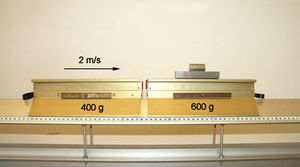Air cushion track with two gliders
1. A glider moves at a speed of 1.5 m / s towards a stationary glider of the same weight. A Velcro fastener ensures that the gliders stay together after the impact. The joint speed of the gliders after the impact is 0.75 m / s. Further experiments show that the speed at a push with Velcro, which one inelastic calls, is always halved.
2. If you double the mass of the approaching glider, the combined speed increases to 1 m / s. If you double the mass of the initially stationary slider, the speed after the inelastic collision is only 0.5 m / s.
3. If the two gliders are allowed to move towards each other at exactly the same speed in opposite directions, they will stop after the inelastic collision.
4. If a spring is tensioned between two sliders by means of two threads, the two sliders move apart at opposite speeds as soon as the threads are separated.

### Impulse: sign and unit

In the first experiment, we applied a certain amount of momentum or impulse to the glider by hand. The glider then maintained its momentum until it hit the impact partner. In the push, the glider transmitted half of its momentum to the partner, which halved the speed of the former. If you double the mass of the approaching glider, it retains 2/3 of its content after the impact, which means that the speed only drops by a third from 1.5 m / s to 1 m / s. If the mass of the resting body is doubled, it takes over 2/3 of the momentum and the speed drops to 0.5 m / s. In summary, we can say that the impulse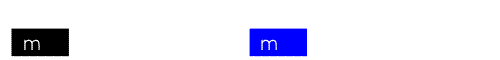• is an accountable maintenance quantity
• forces the movement of a body (no movement without impulse)
• grows proportionally with speed
• is proportional to the mass of the storage body

Had this idea Christiaan Huygens as early as the 17th century. But at that time nobody knew an explanation for experiments 3 and 4. How can nothing be left when two sets flow together, i.e. how can two sets add up to zero? And how do you explain the movement of the two gliders under the action of a spring that itself had no momentum? Such processes are really difficult to explain. In the case of the electric charge, it took about a hundred years before the idea of ​​a quantity capable of sign was accepted. The impetus is only found in connection with quantum mechanics, i.e. not until 200 years later Isaac Newtons Publications on mechanics, accepted as a balance sheet quantity.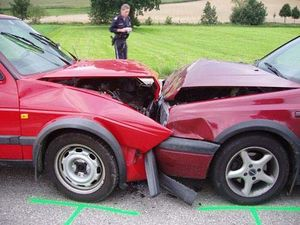Two cars collide head-on. During the impact, the impulse passes through the crumple zones from one car to the other. Because one car had an excess of momentum and the other a lack of momentum, both vehicles no longer show any momentum after the impact. But which car previously had an excess of momentum and which one a deficiency? As with the electric charge, this question of sign can only be answered with the help of a convention. In mechanics, this agreement is of a purely geometric nature. With every problem you have to define by marking a direction when a body has an excess of momentum and when it has a deficiency:

• If a body moves forward, it has an excess of momentum
• If there is a lack of momentum, a body moves backwards, i.e. against the reference direction

The international system of units assigns a coherent unit to the momentum: the momentum content of a body is equal to the product of mass and speed

[math] p_x = mv_x [/ math]

The pulse content (symbol p) is about mass (symbol m) and speed (symbol v) measured. For the unit of momentum we get

[math] [p_x] [/ math] = kgm / s

This measurement specification for the primary variable impulse combines the sign convention for impulse content and speed. A body that has a negative speed, i.e. moves against the positive direction, always shows a lack of momentum. We have already encountered this problem in electrodynamics: one can tell from the potential whether a body is positively or negatively charged. But never forget that the momentum, like the electric charge, is a primary quantity in physics and not just the product of mass and speed.

In the formula above, both momentum and velocity are included x indexed. This index indicates that the momentum discussed here, as well as the associated velocity, are components of vectors. The momentum, like the speed, can thus be broken down into three parts, called components, in relation to the three directions of a fixed-earth coordinate system. Each of these three components is to be accounted for as a separate quantity.

### Fluid image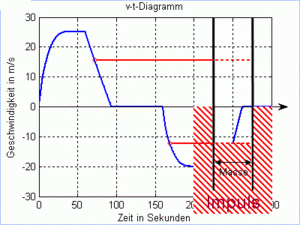Fluid image and v-t-diagram

As in the theory of electricity, we also introduce the fluid image in translational mechanics to illustrate the processes. In the liquid image, every body appears as a pot with the mass as the base and the speed as the filling level: the mass is the capacitive quantity and the speed is the associated potential. In the liquid image, the earth becomes a huge lake in which all the cylindrical pots are lined up.

Now we can visualize a few movement processes

• A body starts moving as soon as an impulse is fed to it from the earth. This process is shown in the liquid picture with a pot (body) to which liquid (impulse) is fed from the large lake. Large pots (bulky bodies) have to absorb a lot of liquid (impulse) in order to reach a certain filling level (speed).
• In order for a body to go backwards, one must withdraw momentum from it and lead it to the earth. In the liquid image, the level in the pot then sinks below that of the lake.
• If two bodies collide, the two pots exchange liquid until their levels have equalized.

The "filling level" of a body is indicated by its speed. If you record the course of the filling level over time, you get the speed-time diagram. This v-t- The diagram has two sides: the dynamic indicates the level of impulse and the kinematic describes the movement of the body. You should memorize the relationship between movement, speed and the appearance of fluids. The speed-time diagram and the fluid image pave the way for the translation mechanics like hardly any other representation.

Examples:

### Bumps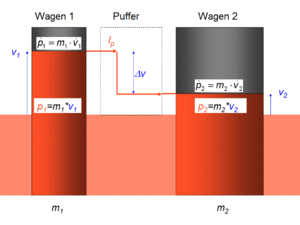Impulse in the event of a shunting impact

Air-cushion vehicles are equipped either with a Velcro fastener or with short, hard springs. If the vehicles collide with the Velcro, they stick together afterwards. The push takes place inelastic. If the vehicles collide with their springs, the impact occurs elastic. Real impacts, such as the impact of one freight wagon against a second, are usually partially elastic. The picture shows the liquid pattern of such a shunting impact.

The impact dynamics can be easily reproduced in the liquid image. To do this, the two cylindrical vessels that represent the vehicles are connected to form a U-tube. In the case of an inelastic collision, the two levels are equal. In the elastic case, the column of liquid overshoots. A real shock is partially elastic. The shock then lies between the inelastic (no overshoot) and the fully elastic limit case (deflection at the end opposite to the deflection at the beginning).

Animations:

Inelastic and fully elastic collisions are quite easy to see through with the help of the liquid image. The real bumps that lie between these two processes give a little more. To do this a

### example

One hovercraft (mass 600 g) collides with a second (mass 400 g) at 2 m / s, which drives towards it at -1 m / s. After the impact, the heavy vehicle comes to a standstill.

1. How fast do the hovercraft move at the moment when they are equally fast?
2. How fast does the light hovercraft move after the impact?
3. How high are the top speeds of the vehicles when the heavier one collides at 3 m / s against the light one that was previously at rest?

### solution

The fluid image is also useful for this task.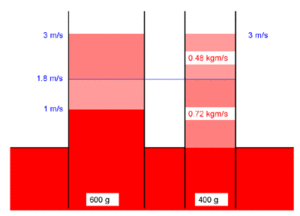1. The total momentum of 0.8 kgm / s (0.6 kg times 2 m / s plus 0.4 kg times -1 m / s) is first evenly distributed over both systems (total mass 1 kg), which results in a combined speed of 0.8 m / s.
2. In the second phase, the heavy vehicle gives another 0.48 kgm / s (0.6 kg times 0.8 m / s) impulse to the light vehicle. This additional momentum transfer increases the speed of the light vehicle by a further 1.2 m / s. As a result, it drives away at 2 m / s.
3. The relative speed of the second impact is the same as that of the first. Only in the second process all speeds are 1 m / s higher than in the first. Therefore, after the impact, both vehicles will each move 1 m / s faster than in the first problem: after the impact, the heavy vehicle has a speed of 1 m / s and the light vehicle glides away at 3 m / s.

### Impulse current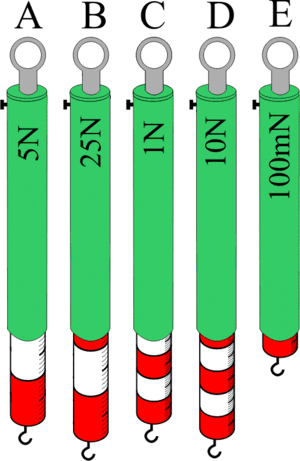A shunting locomotive pulls several cars away, brakes, drives back and then comes to a standstill again (animation). From the liquid image we can see the speed and the momentum of the carriages:

• moving forward: the locomotive pumps impulses from the earth into the train
• braking forwards: The impulse flows away from the train via the locomotive to the earth, releasing energy
• start backwards: the locomotive pumps the impulse away from the train to the earth
• braking backwards: momentum flows into the train, releasing energy from the earth

Let us now turn to the pulling and pushing devices

• Driving forward: Impulse flows backwards through the train and the screw couplings are under tension
• brake forwards: impulse flows forward through the train and the buffers are compressed
• start backwards: impulse flows forward through the train and the buffers are compressed
• braking backwards: Impulse flows backwards through the train and the screw couplings are under tension

With this we have found a general rule regarding the impulse currents

In one under train The momentum flows when the spring is standing against the reference direction (backward) and in one under print The momentum flows when the spring is standing in Reference direction (forward)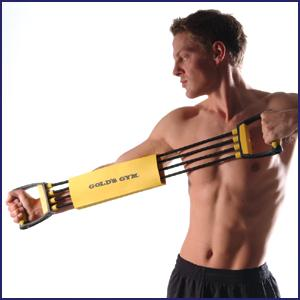According to this rule, the impulse flows around in a circle when tensioning an expander. The impulse flows backwards in the pulled expander and forwards in the compressed chest. The sideways momentum current creates a bend in the arms. Impulse currents that mainly flow in circles can be found everywhere. The engineer calls these momentum flows force flows.

Impulse currents can be measured with scaled springs, so-called spring balances. The unit of the pulse current is Newton (N). The unit of the momentum is therefore equal to the Newton second. The following applies:

[p] = kgm / s = Ns or 1 N = 1 kgm / s2

In electricity, the current is measured in amperes and the charge in ampereseconds. Unfortunately one has not yet introduced a separate unit analogous to Coulomb for the unit of momentum in mechanics. Prof. Friedrich Herrmann from the University of Karlsruhe suggested the Huygens unit for the impulse.

### force

The momentum current is a real transport phenomenon, the force only a measured variable with regard to a selected body

a force is an impulse current with respect to a body

If, for example, two freight wagons collide, the impulse flows from the wagon at the initially higher speed (hammer wagon) into the stationary one (anvil wagon). The associated pulse current can now be measured with respect to the hammer carriage or the anvil carriage. Both currents are shown as oppositely equal force arrows and are referred to as action and reaction force. The following rule applies:

A more flowing Pulse current is measured with an in positive Directional force arrow shown.

### two freight cars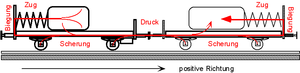A loaded freight car collides with a second one. The indented buffers indicate the strength of the impulse current flowing forward. In the arrangement outlined above, the momentum flows from the load and the mass of the approaching trolley into the anvil trolley and its load. The rule mentioned above can be checked again on the basis of this pulse flow

• Pressure: Impulse flows forward in the car floors and buffers
• Train: Impulse is transported backwards in the two springs
• Shear: momentum flows sideways in the boundary layers between cargo and wagon.

The bend, which is generated by the sideways flowing momentum current, has to do with the transport of angular momentum and will be discussed in a later lecture.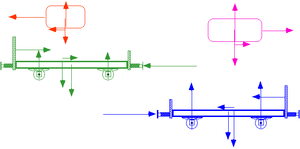If you draw the bodies individually, a force arrow can be assigned to each momentum current. The force arrow points in the positive direction if the impulse flows in. A pulse outflow is shown with an arrow pointing in the negative direction. The length of the force arrow corresponds to the strength of the impulse current. The sketch shows the sectional images of the four bodies. All horizontally directed force arrows belong to the currents of the horizontal impulse. The vertically directed force arrows describe the current strengths of the vertical impulse. Also pay attention to the colors: bodies and associated forces are shown in the same color.

If the impulse flows from one body to a second, two force arrows can always be drawn. The arrow pointing in the negative direction describes the pulse current strength with respect to the first system. The second arrow, which points in the positive direction, corresponds to the pulse inflow with respect to the second system. The two force arrows belonging to a single momentum flow are called an interaction pair (actio equals reactio). Forces are therefore not real objects but only imaginary quantities that are generated in pairs by a cut across a momentum stream. Always compare the force images with the impulse current images! In this way you will learn how momentum current is related to force. You will also find that the direction of the force arrow and the direction of transport of the associated impulse often have nothing to do with each other.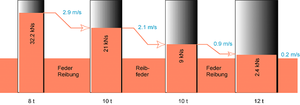The Impulse current pattern shows us how the impulse flows through the body. in the Sectional view the forces, the momentum currents with respect to a selected body, are displayed. As a third representation, we have that Fluid image to disposal. From this picture, which no longer contains the arrangement of the bodies in space, the current filling status of the bodies can be read off.As we will see later, the image of the liquid also provides us with useful information on the energy turnover: whenever the impulse falls in terms of speed, it releases part of the energy it carries with it. When shunting, energy is converted in the two springs, the sliding layers between the load and the wagon and the buffers.

In the future you should ask yourself for each force drawn in any sketch:

• which momentum current is described by this force
• with respect to which system has this impulse been measured

You will be amazed how many power arrows are drawn in incorrectly, senselessly or ambiguously.

### F = m a

The first formulation of mechanics is from Isaac Newton was developed from the motion analysis of the celestial bodies. The sun, planets, moons and comets do not have to be cut free, as they move through space in isolation. But even Newton could not explain how these bodies exchange impulses with one another. Therefore he introduced a gravitational force that cannot be further explained. According to Newton, every celestial body acts on every other according to the product of both masses divided by the square of the distance. This gravitational force is later of Albert Einstein been abolished again. Einstein was able to explain gravitation purely geometrically as the curvature of space-time.

Leonard Euler brought Newton's point mechanics into a mathematical form and extended it to the rigid body. Technical mechanics was later developed from Newton-Euler's theory. With many definitions, additional terms and mathematical theorems, a theoretical building has been developed on the narrow basis of point mechanics that has survived for so long only thanks to the power of habit.

If one assumes the primary quantities momentum and angular momentum, some contaminated sites can be disposed of and a lot can be restructured. The fundamental law of mechanics, Newton's second axiom, can be derived directly from the momentum balance (we have already explained the third axiom, the principle of interaction, above in connection with momentum currents). The momentum balance for a selected body is

[math] \ sum_i I_ {px {_i}} = \ dot p_x [/ math]

The corresponding capacitive law says that the momentum content of a body is equal to mass times speed. If one writes force instead of momentum current strength in relation to a body and inserts the capacitive law into the momentum balance, one receives a comprehensive formulation of the basic law of mechanics

[math] \ sum_i F_x {_i} = m \ dot v_x [/ math]

The sum of all forces is equal to mass times the rate of change in speed. Because the rate of change of speed is called acceleration, the resulting force is equal to mass times acceleration.

In the liquid image, the speed appears as the filling level. Consequently, the acceleration manifests itself as the rate of change in the level or as the "speed" of the liquid level. Newton's basic law only says that the sum of all currents is equal to the base area times the "speed" of the liquid level. You have known this formulation since the first lecture. There, based on Lake Biel, we recorded that the sum of all volume flows is equal to the lake area times the speed of the lake level.

### Control questions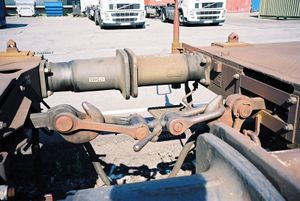1. How do you represent two cars driving towards each other in the liquid image?
2. A car (mass 1400 kg) collides head-on at 18 km / h against a delivery van (3600 kg) approaching at the same speed. Both vehicles wedge together. How fast do the two vehicles move immediately after the impact? How much impulse flows from one vehicle to another during the impact?
3. Can you explain the inelastic collision in the fluid image?
4. What does the elastic shock look like in the liquid image.
5. Railway cars are connected to one another via screw couplings and buffers. When does the impulse flow over the screw coupling and when does it flow over the buffers?
6. With which unit is the pulse current measured? Which unit can one assign to the impulse?
7. What is a surface force?
8. For every force there is an opposing force. What is behind this statement?
9. The sum of all forces is equal to mass times acceleration. Break down this statement, the basic law of mechanics, into a balance equation and a capacitive law.

### Solutions to the control questions

1. In the liquid image, the cars appear as cylindrical pots that stand in a huge lake, which represents the earth. The mass becomes the cross section of the pots and the speed becomes the filling level.
2. The car initially stores 7 kNs and the delivery van -18 kNs of impulse. If the total momentum of -11 kNs is distributed over the total mass of 5000 kg, the result is a speed of -2.2 m / s. During the impact, 10.08 kNs of impulse flow from the car into the delivery van.
3. An inelastic shock appears in the fluid image as a compensation process.
4. An elastic shock is represented as a frictionless overshoot.
5. The pulse flows forwards in the buffers and backwards in the coupling (with respect to a direction that is assumed to be positive).
6. The impulse current is measured in Newtons (N). Hence the momentum can be given in Newton-Second (Ns).
7. A surface force is an impulse current with respect to a body.
8. For every outflow from one system there is an inflow into a second.
9. Newton's principle of action, also called the Basic Law of Mechanics, actually consists of three parts
1. Momentum balance: [math] \ sum_i F_i = \ dot p [/ math]
2. Capacitive law: [math] p = mv [/ math]
3. Kinematic definition of the speed: [math] v = \ dot x [/ math]

### materials

Physics and Systems Science in Aviation 2014

Physics and systems science in aviation